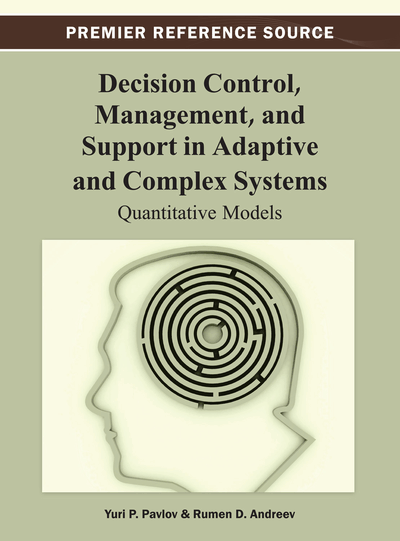# Mathematical Preliminaries

DOI: 10.4018/978-1-4666-2967-7.ch002

## Abstract

The correct assessment of the level of informativity and usability of these types of knowledge requires careful analysis of the terms measurement, formalization, uncertainty, probability, and admissible mathematical operations, under the respective scale, which does not distort the initial empirical information. This chapter is of an introductory nature in one of the vastest fields of mathematics, namely “probability theory and mathematical statistics” and their application in decision-making. After the discussion of fundamental notions and theorems in Probability theory, the authors reveal some of the fundamental techniques establishing the convergence of the recurrent stochastic procedures in the Stochastic programming, which will permit analytical description of the value and utility functions. The analytical description of the expert’s preferences as value or utility function will allow mathematically the inclusion of the decision maker in the description of the complex system “Technologist-process”.
Chapter Preview
Top

## 1. Some Probabilistic Concepts

This chapter is of introductory nature in one of the vastest fields of mathematics, namely “probability theory and mathematical statistics” and their application in decision-making. Since this book is predominantly devoted to methods and adaptive approaches for decision-making under risk and uncertainty conditions, we will begin by introducing the concepts uncertainty and probability with one revelation of the famous Italian scientists de Finetti. De Finetti’s treatise on the theory of probability begins with the provocative statement “probability does not exist,” meaning that probability does not exist in an objective sense. Rather, probability exists only subjectively within the minds of individuals. This opinion of the Italian scientists leads us to philosophical and conceptual thoughts regarding the place of the mathematics in the decision-making process, regarding our capabilities for perceiving the surrounding reality and our ability to reflect it as knowledge and skills for altering our living environment. This revelation leads us far in new horizons before the human thinking and the creative capacity.

There are different approaches with respect to the uncertainty in the human preferences in the decision-making process. The process itself is classified in different ways, individual or group decisions making, decision-making under determinacy or risk, descriptive or normative approach for decision-making, etc.

When the decision-making process is described mathematically, it is interpreted as a mathematical problem within the framework of a particular mathematical theory. At this stage, a conceptual correspondence between the real evaluated processes and the axiomatic foundation of the respective theory is sought. Here the leading role is played by the professional knowledge, intuition, as well as ability to construct adequate theory or models, reflecting the investigated phenomenon. At the next stage, depending on the available information or the ability to encompass the different aspects of the described or investigated processes we introduce the classification “in conditions of determinacy or risk.” We say that we make decisions in condition of deterministic situation if each action leads to unambiguous outcome. Here as a fundamental mathematical apparatus may be used linear and non-linear programming in their different aspects, as part of the vast mathematical discipline called “Operations research” (Bazara, 1979; Zaichenko, 1988). The decision-making in deterministic situation, in mathematical sense, consists of finding an extremum of certain index in an adequate mathematical model of the real process. The right choice of this index in accordance with the available information and measurement scales is crucial. If this stage is missed and the types of measurement scales are not taken into account during the modeling and the decision-making, then the whole process of mathematical modeling and decision may be faulty (Pfanzagl, 1971).

In lesser nominal scales, for instance, scales of ordinal order combinatorial models and algorithms are used. Often time the only optimization algorithms here are of general nature, of the “branch and bound” type. At this stage of modeling and decisions there are difficulties not only of computational kind, but also of conceptual nature. For example, here is the place of the famous Arrow’s theorem, also known as Arrow’s Paradox (Litvak, 1982; Ekeland, 1979).

In greater scales, as the interval scale and ratio scale, until recently there were no strictly proven analytical methods for practical application of the famous theorems for existence in the theory of measurement and the utility theory. Here, to a greater extent are known algorithms described in the books of the famous scientists Keeny and Raiffa, which contain elements of empiricism and are more likely associatively transferred from the representation theorems in utility theory (Keeney, 1993; Raiffa, 1968). With respect to their application aspect, there are often debates and objections reflected in the well known “Allais Paradox” and in the research of many scientists like Khanneman, Tversky, Machina, etc (Kahneman, 1979; Machina, 1982; Mengov, 2010). Obviously a mathematical theory that will establish strictly numerical methods for practical application and the use of the theoretical advancements in the field of mathematical decision-making theory, represented most vividly in the theory of measurement and utility theory, is needed.

## Complete Chapter List

Search this Book:
Reset